# MLF - Balancing Data and Confusion Matrix

Last updated: September 7th, 2020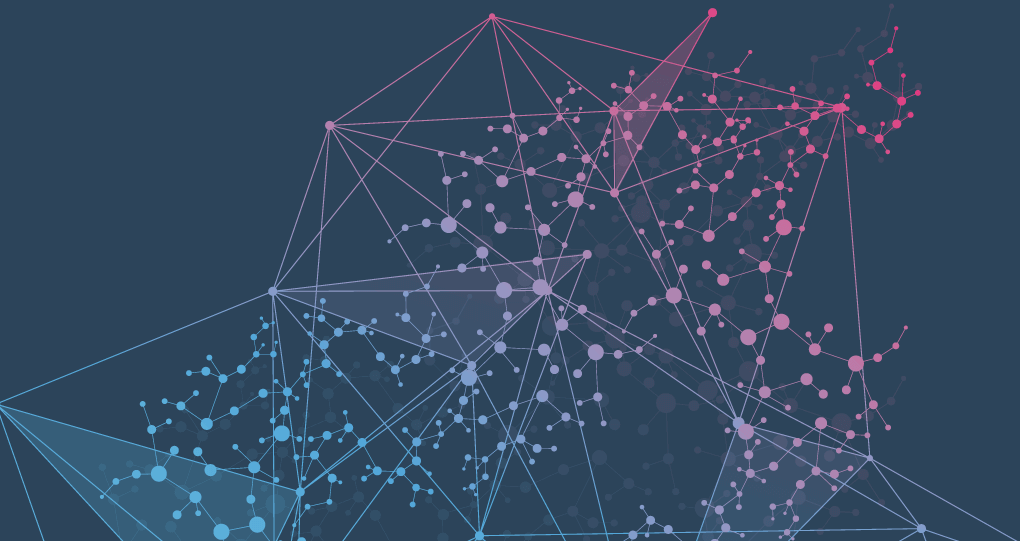# Balancing data and Confusion Matrix¶

In this lesson we will continue the machine learning application from previous lesson. In the process, we will introduce some machine learning core concepts and terms.## Remember the problem¶

Over the past few years, streaming services with huge catalogs have become the primary means through which most people listen to their favorite music. But at the same time, the sheer amount of music on offer can mean users might be a bit overwhelmed when trying to look for newer themes that suits their tastes.For this reason, streaming services have looked into means of categorizing music to allow for personalized recommendations. One method involves direct analysis of the raw audio information in a given song, scoring the raw data on a variety of metrics.

In this lesson we'll be examining data compiled by a research group known as The Echo Nest.

Our goal is to look through this dataset and classify songs as being either 'Hip-Hop' or 'Rock' - all without listening to a single one ourselves. In doing so, we will learn how to clean our data and do some exploratory data visualization towards the goal of feeding our data through a simple machine learning algorithm.## Get the data and our latest model¶

We will get tracks data as we left it in previous lesson, and use it to train a KNeighborsClassifier model as we did before.

In :
import numpy as np
import pandas as pd
import matplotlib.pyplot as plt

tracks = pd.read_csv('tracks_2.csv')

In :
tracks.head()

Out:
track_id acousticness danceability energy instrumentalness liveness speechiness tempo valence genre_top genre_top_code
0 2 0.416675 0.675894 0.634476 0.010628 0.177647 0.159310 165.922 0.576661 Hip-Hop 0
1 3 0.374408 0.528643 0.817461 0.001851 0.105880 0.461818 126.957 0.269240 Hip-Hop 0
2 5 0.043567 0.745566 0.701470 0.000697 0.373143 0.124595 100.260 0.621661 Hip-Hop 0
3 134 0.452217 0.513238 0.560410 0.019443 0.096567 0.525519 114.290 0.894072 Hip-Hop 0
4 153 0.988306 0.255661 0.979774 0.973006 0.121342 0.051740 90.241 0.034018 Rock 1
In :
tracks.info()

<class 'pandas.core.frame.DataFrame'>
RangeIndex: 4802 entries, 0 to 4801
Data columns (total 11 columns):
#   Column            Non-Null Count  Dtype
---  ------            --------------  -----
0   track_id          4802 non-null   int64
1   acousticness      4802 non-null   float64
2   danceability      4802 non-null   float64
3   energy            4802 non-null   float64
4   instrumentalness  4802 non-null   float64
5   liveness          4802 non-null   float64
6   speechiness       4802 non-null   float64
7   tempo             4802 non-null   float64
8   valence           4802 non-null   float64
9   genre_top         4802 non-null   object
10  genre_top_code    4802 non-null   int64
dtypes: float64(8), int64(2), object(1)
memory usage: 412.8+ KB


#### Select Features ($X$) and Labels ($y$)¶

In :
X = tracks.drop(['track_id', 'genre_top', 'genre_top_code'], axis=1)
y = tracks['genre_top_code']


#### Train and Test sets¶

In :
from sklearn.model_selection import train_test_split

X_train, X_test, y_train, y_test = train_test_split(X, y,
test_size=0.2,
random_state=10)


#### Data normalization¶

In :
from sklearn.preprocessing import StandardScaler

scaler = StandardScaler()

In :
scaler.fit(X_train)

X_train = scaler.transform(X_train)
X_test = scaler.transform(X_test)


#### Build and train the model¶

In :
from sklearn.neighbors import KNeighborsClassifier

k=5
model = KNeighborsClassifier(n_neighbors=k)

In :
model.fit(X_train, y_train)

Out:
KNeighborsClassifier(algorithm='auto', leaf_size=30, metric='minkowski',
metric_params=None, n_jobs=None, n_neighbors=5, p=2,
weights='uniform')

#### Make predictions¶

In :
y_pred = model.predict(X_test)


#### Evaluate the model¶

In :
from sklearn.metrics import classification_report

model_report = classification_report(y_test, y_pred)

print("Model report: \n", model_report)

Model report:
precision    recall  f1-score   support

0       0.84      0.69      0.76       188
1       0.93      0.97      0.95       773

accuracy                           0.91       961
macro avg       0.89      0.83      0.85       961
weighted avg       0.91      0.91      0.91       961



#### "Precision", "Recall"... what do these values mean?¶## Confusion Matrix¶

If we measure how good our classification model is by the amount of successes it had, only considering the majority class we can be having a false sense that the model works well.

We also need to take a special look at the errors our model made.

In order to understand this a little better, we will use the so-called Confusion Matrix that will help us understand the outputs of our model.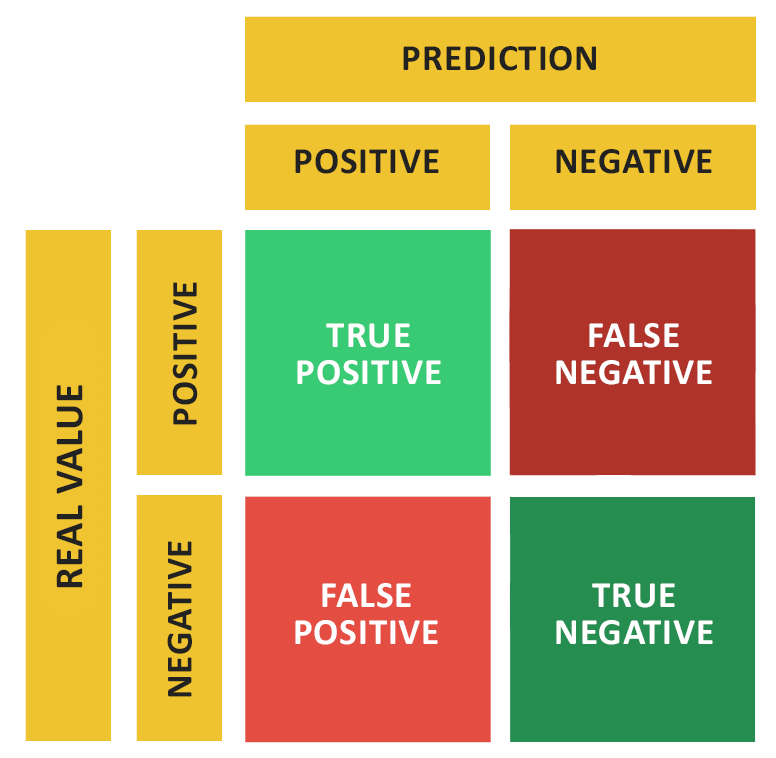There are 4 important terms :

• True Positive (TP): The cases in which we predicted "dog" and the real output was also "dog".
• True Negative (TN): The cases in which we predicted "cat" and the real output was "cat".
• False Positive (FP): The cases in which we predicted "dog" and the real output was "cat".
• False Negative (FN): The cases in which we predicted "cat" and the real output was "dog".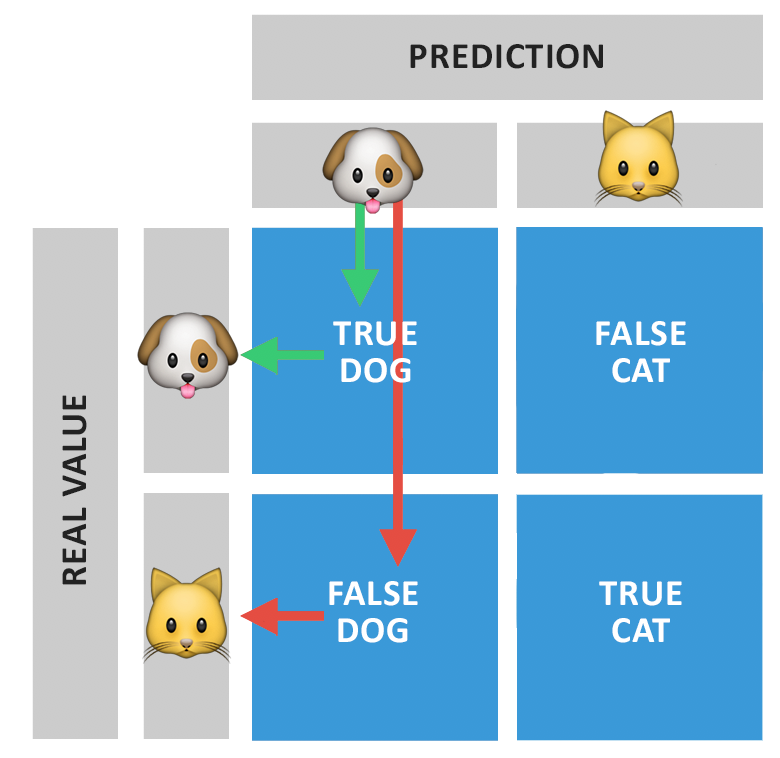In :
from sklearn.metrics import confusion_matrix

conf_matrix = confusion_matrix(y_test, y_pred)
conf_matrix

Out:
array([[130,  58],
[ 24, 749]])

Remember that target values are:

• 0 = Rock
• 1 = Hip-hop
In :
tp, fn, fp, tn = conf_matrix.ravel()
tp, fn, fp, tn

print(f"True positive: {tp} Hip-hop songs were correctly predicted")
print(f"False negative: {fn} Rock songs were wrongly predicted")
print(f"False positive: {fp} Hip-hop songs were wrongly predicted")
print(f"True negative: {tn} Rock songs were correctly predicted")

True positive: 130 Hip-hop songs were correctly predicted
False negative: 58 Rock songs were wrongly predicted
False positive: 24 Hip-hop songs were wrongly predicted
True negative: 749 Rock songs were correctly predicted

In :
import seaborn as sns

plt.figure(figsize=(6,6))
sns.heatmap(conf_matrix, annot=True, cmap="Reds", fmt="d");
plt.title("Confusion matrix")
plt.ylabel('Real values')
plt.xlabel('Predicted values')

Out:
Text(0.5, 33.0, 'Predicted values')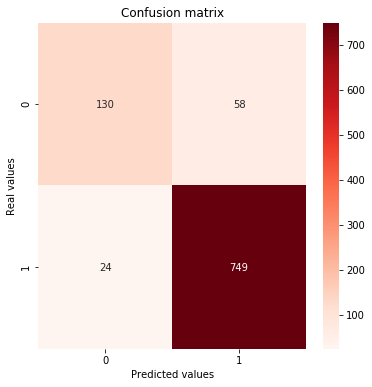EXTRALet's analyze another clear example:

The results of pregnancy tests can have four classifications:

• True positive: a woman is pregnant and is predicted as pregnant
• True negative: a woman is not pregnant and is predicted as not pregnant
• False positive: a woman is not pregnant but is predicted as pregnant, also known as a ‘Type 1’ error
• False negative: a woman is pregnant but is predicted as not pregnant, also known as a ‘Type 2’ error

And from here come new metrics:

### Accuracy¶

Classification Accuracy is what we usually mean, when we use the term accuracy.

The Accuracy of the model is basically the total number of correct predictions divided by the total number of predictions.

From 0 (worst) to 1 (best).

$$Accuracy = \frac{True Positive + True Negative}{Total\ number\ of\ predictions\ made}$$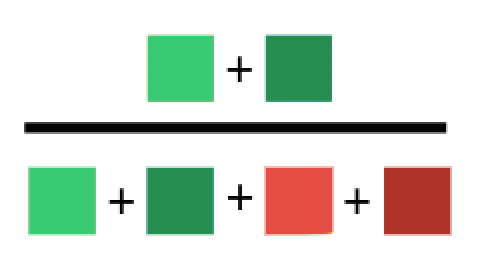Classification Accuracy is great, but gives us the false sense of achieving high accuracy.

### Precision¶

The Precision of a class defines how reliable a model is in responding if a point belongs to that class.

It is the number of correct "positive" results divided by the number of "positive" results predicted.

From 0 (worst) to 1 (best).

Also known as "Positive Predictive Value (PPV)".

$$Precision_{Positive} = \frac{True Positive}{\sum{Prediction\ Positive}}$$$$Precision_{Negative} = \frac{True Negative}{\sum{Prediction\ Negative}}$$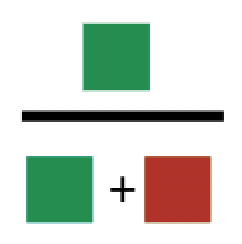### Recall¶

The Recall of a class expresses how well the model can detect that class. Recall values are the one we need to improve, by reducing wrongly predicted values.

It is the number of correct "positive" results divided by the number of all samples that should have been identified as "positive".

From 0 (worst) to 1 (best).

Also known as "Sensibility", "True positive rate (TPR)" or "probability of detection".

$$Recall_{Positive} = \frac{True Positive}{\sum{Real\ Positive}}$$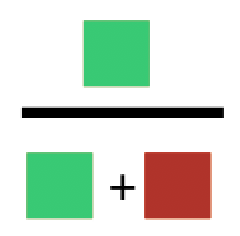$$Recall_{Negative} = \frac{True Negative}{\sum{Real\ Negative}}$$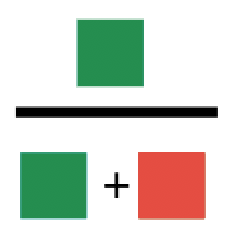### More metrics¶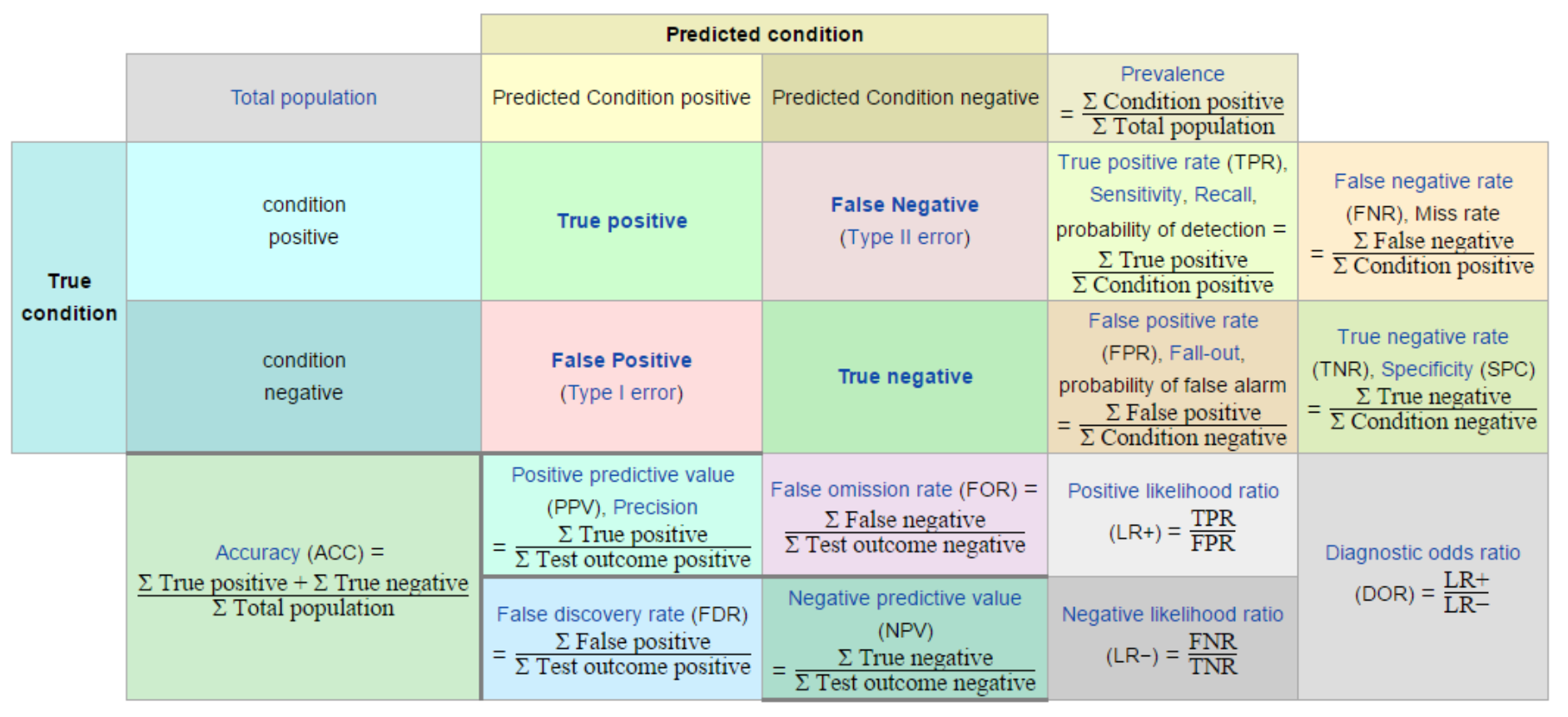Now take a look again at the classification report and calculate manually precision and recall values:

In :
model_report = classification_report(y_test, y_pred)

print("Model report: \n", model_report)

Model report:
precision    recall  f1-score   support

0       0.84      0.69      0.76       188
1       0.93      0.97      0.95       773

accuracy                           0.91       961
macro avg       0.89      0.83      0.85       961
weighted avg       0.91      0.91      0.91       961



#### Precision¶

In :
hiphop_precision = tp / (tp + fp)
print(round(hiphop_precision,2))

0.84

In :
rock_precision = tn / (tn + fn)
print(round(rock_precision,2))

0.93


#### Recall¶

In :
hiphop_recall = tp / (tp + fn)
print(round(hiphop_recall,2))

0.69

In :
rock_recall = tn / (tn + fp)
print(round(rock_recall,2))

0.97


### What should we do with these values?¶

We have four possible cases for each class:

• High precision and high recall: the model handles that class perfectly.
• High precision and low recall: the model does not detect the class very well, but when it does it is highly reliable.
• Low accuracy and high recall: The class detects the class well but also includes samples from other classes.
• Low accuracy and low recall: The model fails to classify the class correctly.

When we have a dataset with imbalanced data, we will obtain a high precision value in the Majority class and a low recall in the Minority class.## Data distribution¶

As we said before, the genre_top variable will be our target variable, and we can see Rock songs clearly outweighs the hip-hop counterpart.

Values are not balanced, we have too many more Rock than Hip-Hop songs.

In :
tracks['genre_top'].value_counts()

Out:
Rock       3892
Hip-Hop     910
Name: genre_top, dtype: int64
In :
tracks['genre_top'].value_counts().plot(kind='bar', figsize=(14,6))

Out:
<matplotlib.axes._subplots.AxesSubplot at 0x7f85fa635820>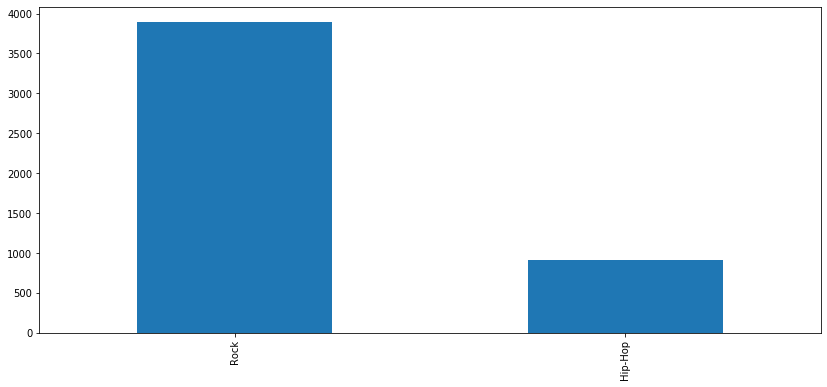## Balancing data¶

We have far more data points for the rock classification than for hip-hop, potentially skewing our model's ability to distinguish between classes.

This is a surprisingly common problem in machine learning (specifically in classification), occurring in datasets with a disproportionate ratio of observations in each class.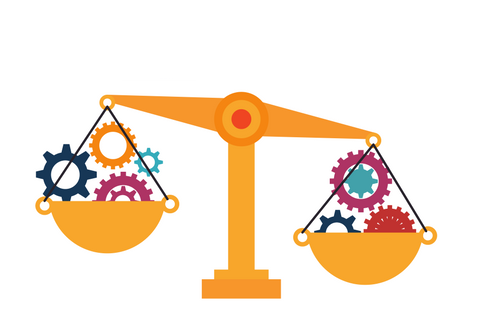This problem usually affects algorithms in their process of generalization of information and harm minority classes.

To solve this, we will down-sample the majority class by randomly removing Rock observations to prevent its signal from dominating the learning algorithm.

The most common heuristic for doing so is resampling without replacement.

### Step 1¶

First, we'll separate observations from each class into different DataFrames.

In :
rock_only = tracks[tracks['genre_top'] == 'Rock']
hip_hop_only = tracks[tracks['genre_top'] == 'Hip-Hop']

In :
rock_only.shape

Out:
(3892, 11)
In :
hip_hop_only.shape

Out:
(910, 11)

We will make a copy of the original dataset

In :
original=tracks.copy()


### Step 2¶

We'll resample the majority class (Rock) without replacement, setting the number of samples to match that of the minority class.

• replace=False indicates that the sample will be without replacement.
• n_samples=hip_hop_only.shape indicates that we will match the size of the minority class, Hip-Hop.
• random_state=1 we define a seed to be able to reproduce this resample results.
In :
from sklearn.utils import resample

rock_downsampled = resample(rock_only,
replace=False,
n_samples=hip_hop_only.shape,
random_state=1)


### Step 3¶

Finally, we'll combine the down-sampled majority class DataFrame with the original minority class DataFrame.

In :
tracks = pd.concat([rock_downsampled, hip_hop_only])

In :
tracks['genre_top'].value_counts()

Out:
Rock       910
Hip-Hop    910
Name: genre_top, dtype: int64
In :
tracks['genre_top'].value_counts().plot(kind='bar', figsize=(14,6))

Out:
<matplotlib.axes._subplots.AxesSubplot at 0x7f85ff3d9a60>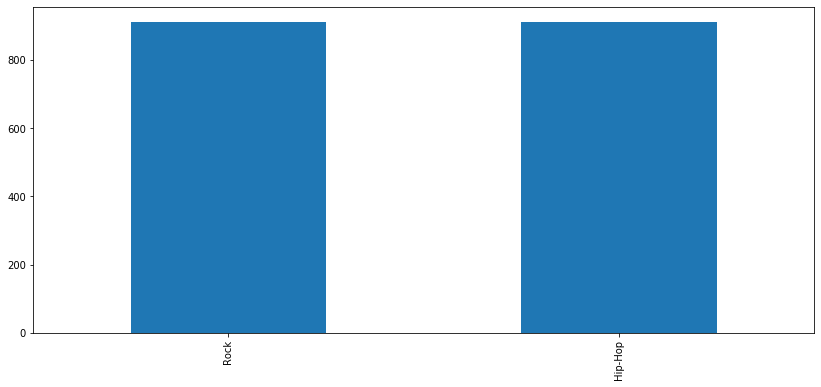We've now balanced our dataset, but in doing so, we've removed a lot of data points that might have been crucial to training our models.## Modeling with the balanced data¶

We will keep using a k-nearest neighbors classifier.

Let's test to see if balancing our data improves model bias towards the "Rock" classification while retaining overall classification performance.

#### Select Features ($X$) and Labels ($y$)¶

In :
X = tracks.drop(['track_id', 'genre_top', 'genre_top_code'], axis=1)
y = tracks['genre_top_code']


#### Split the dataset¶

In :
X_train, X_test, y_train, y_test = train_test_split(X, y,
test_size=0.2,
random_state=10)


#### Data normalization¶

In :
from sklearn.preprocessing import StandardScaler

scaler = StandardScaler()

In :
scaler.fit(X_train)

X_train = scaler.transform(X_train)
X_test = scaler.transform(X_test)


#### Build and train the model¶

In :
k=5
model = KNeighborsClassifier(n_neighbors=k)

In :
model.fit(X_train, y_train)

Out:
KNeighborsClassifier(algorithm='auto', leaf_size=30, metric='minkowski',
metric_params=None, n_jobs=None, n_neighbors=5, p=2,
weights='uniform')

#### Make predictions¶

In :
y_pred = model.predict(X_test)


Let's visually analize predictions and errors:

In :
# select two columns
axis_1 = X_test[:, 1] # danceability column
axis_2 = X_test[:, 4] # liveness column

In :
# define blue and green colors for predictions
pred_colors = ['#27ae60' if pred == 0 else '#2980b9' for pred in y_pred]

In :
plt.figure(figsize=(14,6))

plt.scatter(axis_1, axis_2, s=30, alpha=0.9, c=pred_colors)
plt.xlabel('danceability')
plt.ylabel('acousticness')

Out:
Text(0, 0.5, 'acousticness')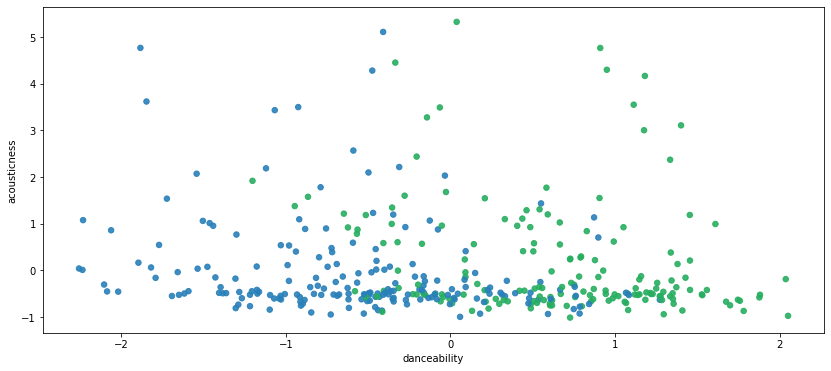In :
plt.figure(figsize=(14,6))

plt.scatter(axis_1, axis_2, s=30, alpha=0.3, c=pred_colors)
plt.xlabel('danceability')
plt.ylabel('acousticness')

# plot a cross on wrong predictions
wrong_pred = y_pred != y_test
plt.scatter(axis_1[wrong_pred], axis_2[wrong_pred], s=40, marker='x', c='#e74c3c')

Out:
<matplotlib.collections.PathCollection at 0x7f85f8c83fa0>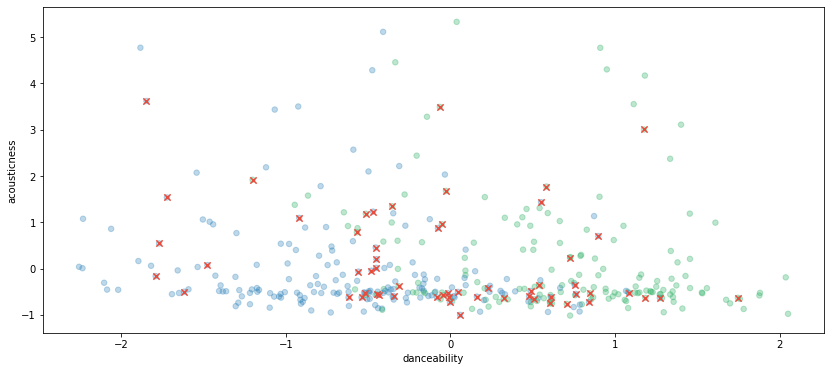#### Evaluating the Model¶

Let's get the test set score:

In :
print("Test set score: {:.2f}".format(np.mean(y_pred == y_test)))

Test set score: 0.84


Also, create a classification report:

In :
model_report = classification_report(y_test, y_pred)

print("Model report: \n", model_report)

Model report:
precision    recall  f1-score   support

0       0.87      0.80      0.84       184
1       0.81      0.88      0.84       180

accuracy                           0.84       364
macro avg       0.84      0.84      0.84       364
weighted avg       0.84      0.84      0.84       364



As we can see, by balancing our data, we improve model bias and now "Rock" and "Hip-Hop" classes have almost the same prediction accuracy.

Recall values goes up.

Overall classification performance was reduced.

In :
conf_matrix = confusion_matrix(y_test, y_pred)

plt.figure(figsize=(6,6))
sns.heatmap(conf_matrix, annot=True, cmap="Reds", fmt="d");
plt.title("Confusion matrix")
plt.ylabel('Real values')
plt.xlabel('Predicted values')

Out:
Text(0.5, 33.0, 'Predicted values')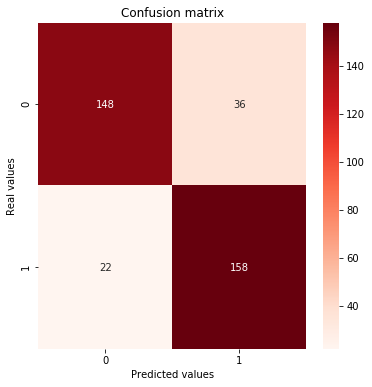## Important Note¶

Always split into test and train sets BEFORE trying oversampling or undersample techniques! Re-sampling before splitting the data can allow the exact same observations to be present in both the test and train sets. This can allow our model to simply memorize specific data points and cause overfitting and poor generalization to the test data.

Let's make our analysis again re-sampling after splitting the dataset

#### Split the dataset¶¶

In :
X = original
y = original['genre_top_code']

In :
X_train, X_test, y_train, y_test = train_test_split(X, y,
test_size=0.2,
random_state=10)


#### Re-sample the training dataset¶

In :
rock_only = X_train[X_train['genre_top'] == 'Rock']
hip_hop_only = X_train[X_train['genre_top'] == 'Hip-Hop']

In :
rock_downsampled = resample(rock_only,
replace=False,
n_samples=hip_hop_only.shape,
random_state=1)

In :
train_balance = pd.concat([rock_downsampled, hip_hop_only])


#### Drop extra features¶

In :
X_train = train_balance.drop(['track_id', 'genre_top', 'genre_top_code'], axis=1)
y_train = train_balance['genre_top_code']

X_test= X_test.drop(['track_id', 'genre_top', 'genre_top_code'], axis=1)


#### Data normalization¶

In :
scaler = StandardScaler()
scaler.fit(X_train)

X_train = scaler.transform(X_train)
X_test = scaler.transform(X_test)


#### Build and train the model¶

In :
k=5
model = KNeighborsClassifier(n_neighbors=k)

In :
model.fit(X_train , y_train)

Out:
KNeighborsClassifier(algorithm='auto', leaf_size=30, metric='minkowski',
metric_params=None, n_jobs=None, n_neighbors=5, p=2,
weights='uniform')

#### Evaluate the model¶

In :
y_pred = model.predict(X_test)

In :
model_report = classification_report(y_test, y_pred)

print("Model report: \n", model_report)

Model report:
precision    recall  f1-score   support

0       0.65      0.82      0.72       188
1       0.95      0.89      0.92       773

accuracy                           0.88       961
macro avg       0.80      0.86      0.82       961
weighted avg       0.89      0.88      0.88       961



The metrics (precision, recall and f1-score) are lower for Hip-Hop than the previous analysis, but also more realistic. It is important to evaluate the model with a dataset that present a similar distribution of classes that the original data.